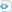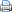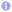C++

# Floating-point exceptions

During a numerical calculation various kinds of run-time errors may occur. In C++, such an error may be indicated via floating-point exceptions or via the (global but thread-local) variable errno. Floating-point exceptions are completely unrelated to C++ exceptions. When an floating-point exception is raised by an erroneous operation, the exception is simply noted in the floating-point status word, and the program continues. The operation produces a default value, which depends on the exception (see the table below). C++11 introduces with the new header file cfenv functions to check and to manipulate the floating-point environment and the floating-point status flags. In this way, a program can find out which exceptions have been raised. The value of the macro math_errhandling indicates the type of error handling that is performed by the floating-point operators and functions.

The IEEE 754 standard defines five floating-point exceptions:

• Invalid operation, e.g., square root of a negative number, which returns quiet NaN by default.
• Division by zero, i.e., an operation on finite operands gives an exact infinite result, e.g., $1/0$ or $\log(0)$), which returns $\pm\infty$ by default.
• Overflow, i.e., a result that is too large to be represented correctly by a floating-point number, which returns $\pm\infty$ by default (for round-to-nearest mode).
• Underflow, i.e., a result that is very small (outside the normal range) and is inexact, which returns a denormalized value by default.
• Inexact, which returns correctly rounded result by default.

The following program raises various floating-point exceptions and illustrates how to detect them. First, one has to tell the compiler that the floating-point environment will be investigated via the #pragma STDC FENV_ACCESS ON, which forces the compiler to prevent some optimizations, which may invalidate the floating-point status. The function feclearexcept clears the specified floating-point status flags, while fetestexcept determines which of the specified floating-point status flags are set. Floating-point exceptions are represented by macro constants FE_INVALID etc. The program’s expected output is:

MATH_ERRNO is set
MATH_ERREXCEPT is set

1.0/0.0 = inf
ERROR: division by zero

2^2000 = inf
ERROR: numerical overflow

1/2^2000 = 0
ERROR: numerical underflow

sqrt(-1) = -nan
ERROR: invalid result


 Testing for floating-point exceptions.#pragma STDC FENV_ACCESS ON

#include <cstdlib>
#include <iostream>
#include <cfenv>
#include <cmath>

void test_fp_exceptions() {
bool error=false;
if (std::fetestexcept(FE_DIVBYZERO)) {
error=true;
std::cerr << "ERROR: division by zero\n";
}
if (std::fetestexcept(FE_OVERFLOW)) {
error=true;
std::cerr << "ERROR: numerical overflow\n";
}
if (std::fetestexcept(FE_UNDERFLOW)) {
error=true;
std::cerr << "ERROR: numerical underflow\n";
}
if (std::fetestexcept(FE_INVALID)) {
error=true;
std::cerr << "ERROR: invalid result\n";
}
if (not error)
std::cerr << "no error\n";
std::feclearexcept(FE_ALL_EXCEPT);
std::cerr << '\n';
}

int main() {
double zero=0.0;

std::cout << "MATH_ERRNO is "
<< (math_errhandling & MATH_ERRNO ? "set" : "not set") << '\n'
<< "MATH_ERREXCEPT is "
<< (math_errhandling & MATH_ERREXCEPT ? "set" : "not set") << '\n'
<< '\n';

std::feclearexcept(FE_ALL_EXCEPT);

std::cout <<  "1.0/0.0 = " << 1.0/zero << '\n';
test_fp_exceptions();

std::cout << "2^2000 = " << std::pow(2., 2000) << '\n';
test_fp_exceptions();

std::cout << "1/2^2000 = " << std::pow(2., -2000) << '\n';
test_fp_exceptions();

std::cout << "sqrt(-1) = " << std::sqrt(-1.) << '\n';
test_fp_exceptions();

return EXIT_SUCCESS;
}

Testing  explicitly for floating-point exceptions can become tedious. The GNU C library provides the functions feenableexcept, fedisableexcept, and fegetexcept, to enable (and disable) traps for floating-point exceptions. This means, a floating-point exception is turned into a signal, which may be caught by a signal handler, as shown by the following program, which requires the GNU C++ compiler to compile.

 Turning a floating-point exception into a signal.#pragma STDC FENV_ACCESS ON
#include<cstdlib>
#include<iostream>
#include<cfenv>
#include<fenv.h>
#include<signal.h>

void floating_point_handler(int signal, siginfo_t *sip, void *uap) {
std::cerr << "floating point error at " << sip->si_addr << " : ";
int code=sip->si_code;
if (code==FPE_FLTDIV)
std::cerr << "division by zero\n";
if (code==FPE_FLTUND)
std::cerr << "underflow\n";
if (code==FPE_FLTOVF)
std::cerr << "overflow\n";
if (code==FPE_FLTINV)
std::cerr << "invalid result\n";
std::abort();
}

int main() {
std::feclearexcept(FE_ALL_EXCEPT);
feenableexcept(FE_DIVBYZERO | FE_UNDERFLOW | FE_OVERFLOW | FE_INVALID);
struct sigaction act;
act.sa_sigaction=floating_point_handler;
act.sa_flags=SA_SIGINFO;
sigaction(SIGFPE, &act, NULL);

double zero=0.0;
double one=1.0;

std::cout << "1.0/1.0 = " << one/one << '\n';
std::cout << "1.0/0.0 = " << one/zero << '\n';
std::cout << "1.0/1.0 = " << one/one << '\n';

return EXIT_SUCCESS;
}

Usually a signal handler will not do much more than emitting an error message, performing some clean-up work, and aborting the program, in particular, when the floating-point error is fatal, e.g., division by zero. Furthermore, when the program exits the signal handler, the floating point-exception is raised again. Consequently, the signal handler is called again, which results into an infinite loop of calls to the signal handler. A solution to this problem is to employ non-local jumps via sigsetjmp and siglongjmp to implement an exception mechanism, as illustrated below.

 title#pragma STDC FENV_ACCESS ON

#include<cstdlib>
#include<iostream>
#include<cfenv>
#include<fenv.h>
#include<signal.h>
#include<setjmp.h>

static sigjmp_buf jmpbuf;

void floating_point_handler(int signal, siginfo_t *sip, void *uap) {
std::cerr << "floating point error at " << sip->si_addr << " : ";
int code=sip->si_code;
if (code==FPE_FLTDIV)
std::cerr << "division by zero\n";
if (code==FPE_FLTUND)
std::cerr << "underflow\n";
if (code==FPE_FLTOVF)
std::cerr << "overflow\n";
if (code==FPE_FLTINV)
std::cerr << "invalid result\n";
feenableexcept(FE_DIVBYZERO | FE_UNDERFLOW | FE_OVERFLOW | FE_INVALID);
siglongjmp(jmpbuf, 1);
}

int main() {
std::feclearexcept(FE_ALL_EXCEPT);
feenableexcept(FE_DIVBYZERO | FE_UNDERFLOW | FE_OVERFLOW | FE_INVALID);
struct sigaction act;
act.sa_sigaction=floating_point_handler;
act.sa_flags=SA_SIGINFO;
sigaction(SIGFPE, &act, NULL);

double zero=0.0;
double one=1.0;

if (sigsetjmp(jmpbuf, 1)==0) {  // try
std::cout << "1.0/1.0 = " << one/one << '\n';
std::cout << "1.0/0.0 = " << one/zero << '\n';
std::cout << "1.0/1.0 = " << one/one << '\n';
} else {  // catch
std::cerr << "some error occurred\n";
}
return EXIT_SUCCESS;
}

Employing the techniques for catching floating-point exceptions as shown above, erroneous numerical calculations become easy to detect and make it possible to deal with them accordingly.